﻿ Generation and Recombination of a CIGSe Solar Cell under the Influence of the Thickness of a Potassium Fluoride (KF) LayerPublications are Open
Access in this journal
Article Versions
Export Article
• Normal Style
• MLA Style
• APA Style
• Chicago Style
Research Article
Open Access Peer-reviewed

### Generation and Recombination of a CIGSe Solar Cell under the Influence of the Thickness of a Potassium Fluoride (KF) Layer

Djimba Niane , Ousmane Diagne, Alain K. Ehemba, Mouhamadou M. Soce, Moustapha Dieng
American Journal of Materials Science and Engineering. 2018, 6(2), 26-30. DOI: 10.12691/ajmse-6-2-1
Published online: July 18, 2018

### Abstract

In this paper, we study the phenomena of generations and recombination of a CIGSe solar cell under the influence of the thickness of a potassium fluoride (KF) layer. The different thicknesses taken are respectively 15 nm, 30 nm and 45 nm and the doping rate of the base has been fixed at 1016cm-3. The simulations made with the SCAPS-1D software show, on the one hand, a decrease in the emission rate and an increase in the electron capture rate; on the other hand, an increase in the rate of emission and capture of the holes respectively, as the thickness of the KF layer increases. Furthermore, we note that the rate of recombination of the carriers increases slightly with the thickness of KF at the CIGSe layer caused by a passivation of the defects.

### 1. Introduction

Today the control of photovoltaic energy remains a major stake in the development of society. In this context, the solar cells based CIGSe are those in thin layers that have the best efficiency. The latter continues to increase during the last few months, from 20 to over 22.7 1 thanks to the introduction of post-deposition treatment of potassium fluoride. In this paper, a simulation study is made on the influence of the thickness of KF on the phenomena of generations and recombination. Indeed, in semiconductors as CIGSe, the mechanisms of generations and recombination are linked to the presence of traps. The latter correspond to allow energy levels situated in the forbidden band and have the possibility of trapping an electron or of restoring him. The traps capture and emit free carriers. Consequently, thus they can either occupied by electrons or occupied by holes. A theory describing this effect has been established by Shockley, Read, and Hall 2, 3. Therefore, the effect is throughout the literature referenced as Shockley-Read-Hall (SRH) generation/recombination. Four sub-processes are possible: electron capture, hole capture, hole emission and electron emission

### 2. Numerical Simulation

The structure of a solar cell consists of the stacking of several layers. A substrate (soda-lime glass, polyimide or metal) with a thickness of 1 to 3 mm, is covered with molybdenum (Mo) serving as metal back contact (from a few hundred nanometers to 1 μm). On the latter is deposited the absorbent layer CIGSe thickness of 1 to 3 microns. The CIGSe is heterojunction with a CdS buffer layer 50nm thick and a window layer ZnO of thickness 200nm to 300nm and finally a layer Ni / Al / Ni.

In this work, we study the generation and recombination rates under the influence of a KF layer placed in the CIGSe / CdS interface of a n-ZnO / n-CdS / p-CIGS heterojunction solar cell, represented in Figure 1 at room temperature with SCAPS-1D simulation software 4, 5 under illumination of 1000W / m² under AM1.5 conditions.

• Figure 1. Structure of a heterojunction solar cell n-ZnO/n-CdS/p-CIGS

The properties of the bulk defects of the different layers are presented in Table 1.

The distribution function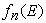represents the probability that a trap is occupied by an electron. Its expression is given by: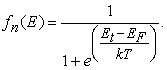(1)

With: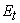is the energy level associated with the defect.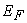the fermi level

√ k the Boltzmann constant

√ T the temperature.

The probability so that this state is not occupied by an electron is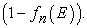This function represents consequently the function of distribution of the holes in the semiconductor. It is expressed in the following way: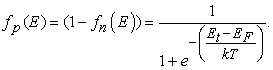(2)

The electron emission rate is determined by knowing the number of places available for an electron in the conduction band. It results from it that the emission of an electron takes place when the electron leaves a neutral trap to go towards the conduction band. Consequently, the emission rate of an electron is expressed as follows: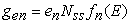(3)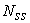is the density of traps and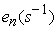is the probability of emission of an electron trapped to the conduction band.

The holes emission rate is equated to the number of electrons leaving the valence band to occupy earlier traps ionized. Taking into account the emission coefficient of the holes by traps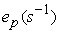, the number of empty trap door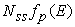, the emission rate of the holes is written: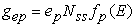(4)

There is capture of an electron when this one leaves the conduction band to go towards to the valence band to occupy a trap ionized earlier. The capture rate of electrons characterizes the fact that an electron that transits is not always trapped. Taking into account the capture probability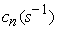, the number of electrons present in the conduction band and the number of unoccupied traps; the electron capture rate per unit volume is expressed by the following relation: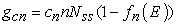(5)

With: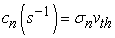Where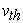represents the thermal velocity of the carriers and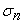the effective cross-section of electron capture by the traps.

The capture of the holes takes place when an electron occupying a trap leaves it to neutralize a hole in the valence band. The capture rate of the holes is proportional to the capture possibilities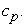Considering the concentration of free holes in the valence band p, the capture rate is expressed as follows: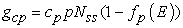(6)

With: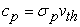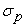is the cross section of capture of the holes by the traps.

### 3. Results and Discussions

Figure 2 illustrates the electron emission rate according of the depth of the CIGSe for different thickness of KF. The doping rate was set at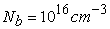for the layer of CIGSe and the different KF taken are respectively 15 nm, 30 nm and 45 nm.

• Figure 2. Emission rate of electrons according of the depth of the CIGSe for different thickness of KF

We find that for base depths between 0 and 1.5μm, the electron emission rate increases, but not significantly. This results in the fact that when the electrons move away from the back contact which constitutes the positive pole of the generator the states of traps start to empty itself. Then for depths between 1.5μm and 2.1μm, we note a decrease in the emissions rate until reaching a minimum. The latter indicates a change accumulation in regime inversion regime because the electrons are approaching the front contact.

Finally, when the depths of the base is 2.1 μm between 2.5 μm, the increase in the emission rate becomes remarkable. This is explained by the considerable increase in the number of electrons at the interface.

In addition, we notice that the emission rate increases slightly with the increase in the thickness of the KF layer. It can be explained by the fact that the increase of the thickness of the layer of KF generates carriers in the layer of CIGSe.

Figure 3 shows the capture rate of electrons as a function of the depth of the base (CIGSe) for different thickness of KF.

We notice that the rate of capture of electrons increases until reach a maximum corresponding to a value of the depth of the layer of CIGSe (in the neighborhood 1.3 μm). This is explained by the considerable increase in the number of free electrons in the conduction band and it becomes very likely to capture an electron. In addition, this capture does not require energy input since the transition is towards the valence band and explains the speed of this mechanism. When the depth of the base is superior to 1.3 μm, the rate of capture decreases until reaching a minimum in the neighborhood of 2.3 μm. This decrease translates the emptying of the traps which accelerates strongly when electrons get closer to the contact before. Another peak is observed in FIG. 3 for depth greater than 2.3 μm explained by an increased presence of defects in the CIGSe / CdS interface.

In addition, we note an increase in the electron capture rate as a function of the thickness of the KF layer. This phenomenon reflects the strong presence of acceptor type defects that accentuate the filling of traps.

Figure 4 shows the holes emission rate as a function of the depth of the base for different thicknesses of the KF layer.

• Figure 4. Emission rate as a function of the depth of the CIGSe for different thickness of the KF layer

Figure 4 shows that the holes emission rate per unit volume remains constant for depth less than 2.3 μm. Indeed, when emitting a hole the electron of the valence band is trapped leaving a hole in the valence band, hence the hole is emitted from the empty trap in the valence band. Given that, it is a process which repeats the issue rate of the holes in the base becomes constant. However, a considerable decrease in the rate is noted when approaching the contact before, in other words when the depth are greater than 2.3μm. The interpretation is made according to which, the issue rate depends amongst hole present. Given that in this zone the number of holes is not important, the reduction in the emission rate becomes inevitable. Moreover, we also notice that the issue rate of the holes almost does not vary with the increase thickness of the layer of KF. However, a small variation is observed when approaching the front contact thus showing an increase in the rate as a function of the thickness of the KF layer. In addition, the largest decrease is reached by the thickness 15nm.

Figure 5 shows the capture rate of the holes as a function of the depth of the base for different thicknesses of the KF layer.

• Figure 5. Capture rate of the holes as a function of the thickness of the CIGSe for different thickness of the KF layer

Figure 5 takes the same forms obtained in figure 3. The physical explanation can be given as follows. Indeed, we know that the electrons move randomly with their thermal velocity and that the G-R centers remain motionless in the CIGSe layer. Nevertheless, it is useful to change reference by considering the immobile electrons (holes) and the G-R centers move at the speed. The centers then sweep a volume per unit of time of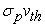. The holes in this volume have a very strong probability of being captured especially since this mechanism does not require a supply of energy.

Carrier generation is a process in which electron-hole pairs are created by exciting an electron from the valence band of the semiconductor to the conduction band, thus creating a hole in the valence band. The emission of electrons and holes respectively are responsible for generations of electron-hole pairs and the excess energy is provided by a lattice.

In its default mode, SCAPS calculates the generation of electron-hole pairs G(x) from the incident photon flux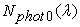from a very coarse optical model. This model contains only reflection /transmission at the two contacts, and the absorption in the layers. From the photon flux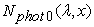at each position and for each wavelength, the generation of eh pairs G(x) is then calculated as: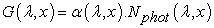(7)

This is then integrated over all wavelengths of the incoming photon flux: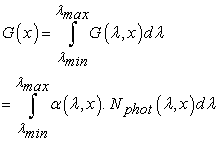(8)

Where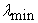and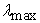are the short and long wavelength limits of the incoming photon spectrumFigure 6 shows the generation rate of the carriers for different thicknesses of the KF layer as function of the depth of cell .

• Figure 6. Generation rate of the carriers for different thicknesses of the KF layer

The analysis of the curve shows a decrease in generation when the thickness of the KF layer is increased. This is supposed to be due to the fact that increasing the thickness of the KF layer leads to recombination of the charge carriers.

Recombination is the reverse process of the generation in which the electrons and holes of the conduction band recombine respectively and are annihilated. The capture of electrons and holes respectively by the traps are responsible for recombination phenomena.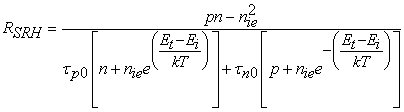(9)

Whereis the energy level associated with the defect,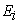is the intrinsic energy level, n, p are the concentration of the electrons and the holes respectively out of equilibrium such that n=n0 +∆n et p= p0 +∆p,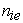the intrinsic concentration, k the Boltzmann constant, T the temperature and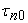,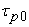the lifetime for the electrons and the holes respectively.

With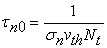(10)

and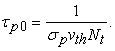(11)

Figure 7 shows the recombination rate of the carriers as function of the depth of cell for different thicknesses of the KF layer.

• Figure 7. Recombination rate of the carriers for different thicknesses of the KF layer

The observation in the Figure 7 shows that the rate of recombination as a function of the thickness of the cell is greater at the level of the buffer layer and that of the window layer when the cell is illuminated. In a solar cell, the recombination rate R depends both on the lifetime of the charge carriers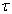and the density of the carriers. In this zone the density of the electrons is more important than that of the holes, it results in a great probability of the carriers to be recombined. We also note that the distribution of the recombination rate is not uniform and the peak is reached in the vicinity of the CIGSe / CdS interface. This is caused by the complex phenomena that exist at the CIGSe / CdS interface such as the existence of grain boundaries and defects 6. Inside the CIGSe layer, we notice that the rate tends to decrease when the carriers come closer to the rear contact. In addition, it appears in Figure 7, particularly at the level of the CIGSe layer that the recombination rate increases as a function of the thickness of the KF layer. This increase is reduced when the carriers pass through the CIGSe layer to collect at the back contact

### 4. Conclusion

Generation and recombination are very important phenomena in the performance of solar cells. In this work, we studied the generation and recombination rates of a CIGSe solar cell under the influence of the thickness of a KF layer. The increase in the thickness of KF causes a decrease in the generation rate but also that of recombination. However, we have noted that the increase in recombination at the CIGSe is not important. These results demonstrate the beneficial effects of incorporating KF into CIGSe growth.

### References

  Philip Jackson , Roland Wuerz, Dimitrios Hariskos, Erwin Lotter, Wolfram Witte, and Michael Powalla, “Effects of heavy alkali elements in Cu(In,Ga)Se2solar cells with efficiencies up to 22.6%”Phys. Status Solidi RRL 10, No. 8, 583-586 (2016). In article View Article  Schockley. W, Read W.T, “Statistics of the Recombinations of Holes and Electrons” Physical Review, 1952, vol 87, n°5, p. 835-842. In article View Article  Hall R. N., “Electron-Hole Recombination in Germanium” Physical Review, 1952, vol 87 n°2 p 387. In article View Article  M. Burgelman, P. Nollet, S. Degrave, “Modelling polycrystalline semiconductor solar cells”, Thin Solid Films 361/362 (2000) pp.527-532. In article View Article  M. Burgelman, K. Decock, S. Khelifi and A. Abass, “Advanced electrical simulation of thin film solar cells”, Thin Solid Films, 535 (2013) 296-301 In article View Article  Mutsumi Sugiyama, Muneatsu Hayashi, Chizuru Yamazaki, Nafisah Binti Hamidon, Yuiko Hirose, Masayuki Itagaki, “Application of impedance spectroscopy to investigate the electrical properties around the pn interface of Cu(In,Ga)Se2 solar cells Application of impedance spectroscopy to investigate the electrical properties around the pn interface of Cu(In,Ga)Se2 solar cells”, Thin Solid Films 535 (2013) 287-290. In article View Article

Published with license by Science and Education Publishing, Copyright © 2018 Djimba Niane, Ousmane Diagne, Alain K. Ehemba, Mouhamadou M. Soce and Moustapha DiengThis work is licensed under a Creative Commons Attribution 4.0 International License. To view a copy of this license, visit http://creativecommons.org/licenses/by/4.0/